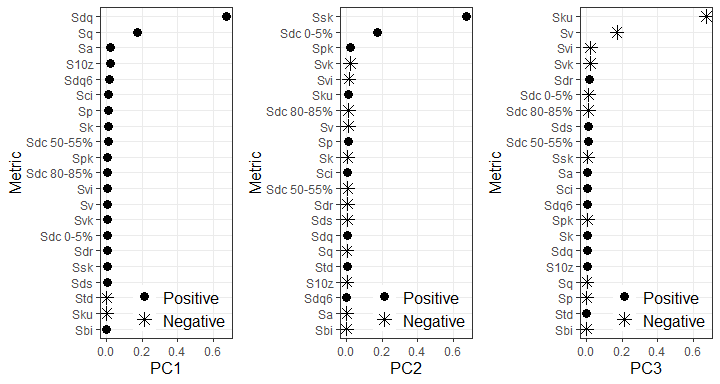geodiv Examples

Learning Objectives

This vignette is designed to:

(1) show how gradient surface metrics are calculated globally and locally using geodiv.

(2) show the potential relationships among metrics.

Example 1, “Simple workflow with Landsat NDVI,” demonstrates the first objective by applying geodiv functions globally and locally to a small region in southwestern Oregon state, USA. Example 2, “Applying all surface metrics across Oregon, USA,” addresses the second objective. The second example applies metrics across a larger region and demonstrates ways in which metrics may be correlated and grouped.

Install geodiv

geodiv is an R package that provides methods for calculating gradient surface metrics for continuous analysis of landscape features. There are a couple of ways to download and install the geodiv R package. You can install the released version of geodiv from CRAN with:

install.packages("geodiv")

And the development version from GitHub with:

install.packages("devtools")
devtools::install_github("bioXgeo/geodiv")

Note that Mac OS users may need to install the development tools here to get the package to install:

https://cran.r-project.org/bin/macosx/tools/

To begin, let’s load the necessary packages for the examples that follow.

library(geodiv)
library(raster)
library(rasterVis)
library(mapdata)
library(maptools)
library(rgeos)
library(ggplot2)
library(tidyverse)
library(parallel)
library(sf)
library(rasterVis)
library(ggmap)
library(corrplot)
library(gridExtra)
library(cowplot)
library(factoextra)
library(cluster)

Example 1: Simple workflow with Landsat NDVI

The National Aeronautics and Space Administration (NASA) has been collecting Earth observing images with Landsat for decades. Normalized Difference Vegetation Index (NDVI) is a measure of vegetation or greenness that can be quantified from Landsat images by measuring the difference between near-infrared and red light, which vegetation strongly reflects and absorbs, respectively. For this first example, we generated an NDVI image over a small region in southwestern Oregon using Google Earth Engine. This image is available as a raster layer available as an R data object entitled ‘orforest’ when geodiv is installed. Google Earth Engine already has a number of tutorials and sample code and functions for this step that can be accessed on its site: earthengine.google.com. There are also several ways in which to access satellite data from R as well. Given that the focus of this tutorial is on the use of the geodiv R package, we provide these data already generated in Google Earth Engine.

Let’s begin by loading the example data, which is a raster layer called ‘orforest’.

data(orforest)
# Check out the properties of the orforest raster layer.
orforest
#> class      : RasterLayer
#> dimensions : 371, 371, 137641  (nrow, ncol, ncell)
#> resolution : 0.0002694946, 0.0002694946  (x, y)
#> extent     : -123, -122.9, 43.00002, 43.1  (xmin, xmax, ymin, ymax)
#> crs        : +proj=longlat +datum=WGS84 +no_defs
#> source     : memory
#> names      : summer_ndvi_p45_r30_2000_2016_30m
#> values     : 0.09595944, 0.8357643  (min, max)

First, we plot the data without any trends removed.

# Plot orforest without the trend removed.
eviCols <- colorRampPalette(c('lightyellow1', 'darkgreen'))(100)
eviTheme <- rasterTheme(region = eviCols)
(orig_ndvi <- rasterVis::levelplot(orforest, margin = F,
par.settings = eviTheme, xlab = 'Longitude',
ylab = 'Latitude', main='orforest original'))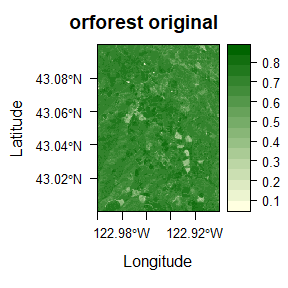Use the ‘remove_plane’ function of geodiv to remove any trend, if present. This function searches polynomials of orders 0 - 3 to determine which is has the lowest error relative to the surface values. To fit a surface with a user-specified polynomial order, you may use the function ‘fitplane.’ Here, the result is a polynomial surface of order 0, so only the mean surface would be removed.

# Remove a polynomial trend.
orfor_rem <- remove_plane(orforest)
#>  "Order of polynomial that minimizes errors: 0"

The simplest use of the geodiv package is to apply metrics globally over an entire image. This returns a measurement of the overall heterogeneity of the image. The ‘sa’ function calculates the average roughness of a surface as the absolute deviation of surface heights from the mean surface height. The ‘sbi’ function calculates the surface bearing index, which is the ratio of the root mean square roughness (Sq) to height at 5% of bearing area (z05). The ‘std’ function calculates texture direction metrics (i.e., the angle of dominating texture and the texture direction of the Fourier spectrum image calculated from the orforest image).

# Calculate global metrics over the entire orforest image.
(sa <- sa(orforest)) # average roughness
#>  0.04466675
(sbi <- sbi(orforest)) # surface bearing index
#>  0.08557302
(std <- std(orforest, create_plot = FALSE, option = 1))
#>  90

Generate texture images

Another common use case is to look at texture images where a spatial function has been applied locally over focal windows across a landscape. This functionality is provided through both the ‘focal_metrics’ and ‘texture_image’ functions.

The ‘texture_image’ function applies metrics in either square or circular windows over an image. This function tends to be faster than ‘focal_metrics’ for large rasters (e.g., > 1000000 pixels), but uses more memory.

The ‘focal_metrics’ function is modified from the ‘window_lsm’ function in landscapemetrics (Hesselbarth, et al. 2019). Windows must be rectangular or square, and the ‘metrics’ argument is a list of functions. The output of this function is a list of rasters. This function is slower than ‘texture_image’ for large rasters, but uses less memory.

For smaller rasters like this example (371x371 pixels), both functions produce the same results in a similar amount of time (~5-15s).

# Texture image creation using 'focal_metrics' function.
window <- matrix(1, nrow = 7, ncol = 7)
system.time(
output_raster <- focal_metrics(orforest, window,
metrics = list('sa'),
progress = TRUE)
)
#>
> Progress metrics:  1 / 1
#>    user  system elapsed
#>    6.27    0.17    6.47

print(output_raster)
#> $sa #> class : RasterLayer #> dimensions : 371, 371, 137641 (nrow, ncol, ncell) #> resolution : 0.0002694946, 0.0002694946 (x, y) #> extent : -123, -122.9, 43.00002, 43.1 (xmin, xmax, ymin, ymax) #> crs : +proj=longlat +datum=WGS84 +no_defs #> source : memory #> names : layer #> values : 0.002336013, 0.202495 (min, max) # Texture image creation using 'texture_image' function. system.time(output_raster2 <- texture_image(orforest, window_type = 'square', size = 7, in_meters = FALSE, metric = 'sa', parallel = FALSE, nclumps = 100)) #>  "mclapply is not supported on Windows, using parLapply instead." #>  "Beginning calculation of metrics over windows..." #> Total time to calculate metrics: 11.57297 #> user system elapsed #> 11.58 0.06 11.64 print(output_raster2) #> class : RasterLayer #> dimensions : 371, 371, 137641 (nrow, ncol, ncell) #> resolution : 0.0002694946, 0.0002694946 (x, y) #> extent : -123, -122.9, 43.00002, 43.1 (xmin, xmax, ymin, ymax) #> crs : +proj=longlat +datum=WGS84 +no_defs #> source : memory #> names : summer_ndvi_p45_r30_2000_2016_30m #> values : 0.002336013, 0.202495 (min, max) Plot the texture image raster. rasterVis::levelplot(output_raster2, margin = F, par.settings = eviTheme, ylab = NULL, xlab = NULL, main = 'Sa')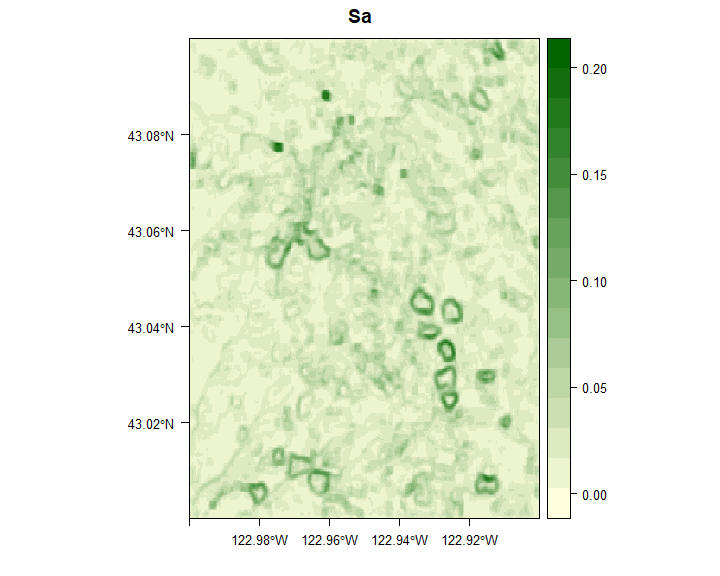Example 2: Applying all surface metrics across the state of Oregon, USA Motivation By assessing heterogeneity using a variety of metrics, researchers can gain a more complete picture of heterogeneity than they would with a single metric (Dahlin, 2016). However, many metrics are related and not all are informative for every situation. To demonstrate the utility of geodiv for producing and evaluating the utility of multiple metrics, in Example 2 we apply all surface metric functions to satellite imagery across Oregon, USA and examine the patterns of, and relationships among, metrics. We calculate metrics for a commonly-used measure of canopy greenness, Enhanced Vegetation Index (EVI), from NASA’s Moderate Resolution Imaging Spectroradiometer (MODIS). We then examine the correlations among metrics along a transect crossing the state, and determine how the metrics cluster using two methods, hierarchical clustering and Principal Components Analysis (PCA). This analysis demonstrates the relationships among metrics, with potential for determining how metrics group and behave with various input data. Note that as with any analysis, researchers will want to use the most appropriate data source and resolution for their own study. The example shown here is only meant as an example to demonstrate the metrics themselves, not to provide data for any subsequent analyses. EVI Data EVI data that are available to use along with this vignette were prepared in Google Earth Engine (Gorelick et al., 2017) and analyzed in R. Void-filled SRTM data aggregated to 240m resolution (Farr et al., 2007) and quality-filtered, maximum growing season, MODIS EVI data at 250m resolution (Didan, 2015) were downloaded in Fall 2019. The code chunk below downloads these data into the current R session from figshare. R may be used to access satellite data, but we provide previously-prepared data for the purpose of this analysis. # Download data from figshare. fs_data <- list("https://ndownloader.figshare.com/files/24366086", "https://ndownloader.figshare.com/files/28259166") # Set timeout option to 1000s to make sure downloads succeed. options(timeout = 1000) # Function to download rasters, including setting a tempfile. get_raster <- function(rasts) { tf <- tempfile() tryCatch(download.file(rasts, destfile = tf, mode = 'wb'), error = function(e) 'File download unsuccessful.') outrast <- raster(tf) return(outrast) } # Download data from figshare. evi <- get_raster(fs_data[]) * 0.0001 Aggregate the raster to ~1km resolution (440640 pixels) for comparison between datasets and to reduce computational time. The most appropriate resolution for any analysis should be based on the study goals and not computational efficiency. Here, we are demonstrating how metrics may vary across space, as well as how metrics relate to one another, and as such have chosen to use a relatively coarse resolution. evi <- raster::aggregate(evi, fact = 4) Pre-processing of rasters Begin by masking any values that are outside of the boundaries for the state of Oregon. state <- maps::map(database = 'state', regions = 'oregon', fill = TRUE, plot = FALSE) statePoly <- map2SpatialPolygons(state, IDs = state$names,
proj4string = CRS(proj4string(evi)))

Generate plots to get a sense for the spatial patterns in the data.

# plot maximum growing season EVI for Oregon
rasterVis::levelplot(evi_masked, margin = F, par.settings = eviTheme,
ylab = NULL, xlab = NULL,
main = 'Maximum Growing Season EVI')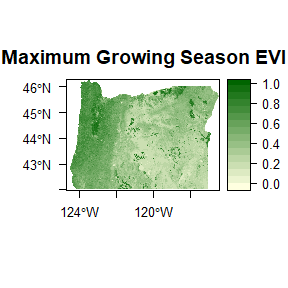Remove any trends in the data with the ‘remove_plane’ function and take a look at the plots of the images with the trends removed.

#>  "Order of polynomial that minimizes errors: 3"

# plot again to see what the new raster looks like
rasterVis::levelplot(evi_masked, margin = F, par.settings = eviTheme,
ylab = NULL, xlab = NULL, main = 'EVI without Trend')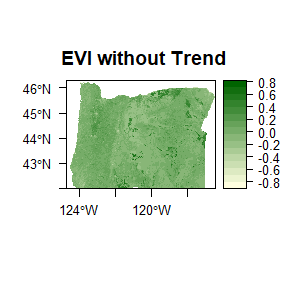Generate texture images of the state of Oregon

Below we generate a texture image for EVI over the state of Oregon using the ‘sa’ metric.

# Calculate EVI 'sa' texture image for state of Oregon.
system.time(outrast <- texture_image(evi_masked, window_type = 'square',
size = 5, in_meters = FALSE, metric = 'sa',
parallel = FALSE, nclumps = 100))
#>  "mclapply is not supported on Windows, using parLapply instead."
#>  "Beginning calculation of metrics over windows..."
#> Total time to calculate metrics: 36.94785
#>    user  system elapsed
#>   36.83    0.35   37.19

We then calculate all metrics over Oregon using the same process. Note that the following step may take some time due to the relatively large image size (440640 pixels). On a computer with 250Gb RAM, and a Intel(R) Xeon(R) Platinum 8260 processor, each texture image took between 15s and up to 2 hours running on 16 cores.

After creating texture images, we convert the raster generated by the ‘texture_image’ function to a dataframe for subsequent analyses. We provide the output dataframe files along with this vignette for all metrics included in geodiv for EVI over 15km x 15km square moving windows and scaled following calculation for the subsequent analyses in this vignette.

Time requirements for both the “texture_image” and “focal_metrics” functions scale linearly with image size, and quadratically with window size. For “texture_image,” more cores leads to lower run times for more complex functions such as “sbi.” However, this is not the case for very simple functions such as “sa,” where fewer cores may be more efficient.

m_list <- list('sa', 'sq', 's10z', 'sdq', 'sdq6', 'sdr', 'sbi', 'sci', 'ssk',
'sku', 'sds', 'sfd', 'srw', 'std', 'svi', 'stxr', 'ssc', 'sv',
'sph', 'sk', 'smean', 'spk', 'svk', 'scl', 'sdc')

outrasts <- list()
system.time(for (i in 1:length(m_list)) { # figure out 16, 24 error
outrasts[[i]] <- texture_image(evi_masked, window_type = 'square',
size = 15, in_meters = FALSE,
metric = m_list[[i]], parallel = TRUE,
nclumps = 100)})
outrasts <- stack(unlist(outrasts))

data_evi <- data.frame(x = coordinates(outrasts)[, 1],
y = coordinates(outrasts)[, 2])
for (i in 1:34) {
data_evi[, i + 2] <- outrasts[[i]][]
}
names(data_evi) <- c('x', 'y', 'Sa', 'Sq', 'S10z', 'Sdq', 'Sdq6', 'Sdr', 'Sbi',
'Sci', 'Ssk', 'Sku', 'Sds', 'Sfd', 'Srw', 'Srwi', 'Shw',
'Std', 'Stdi', 'Svi', 'Str 0.2', 'Str 0.3', 'Ssc', 'Sv',
'Sp', 'Sk', 'Smean', 'Spk', 'Svk', 'Scl min 0.2',
'Scl max 0.2', 'Scl min 0.3', 'Scl max 0.3', 'Sdc 0-5%',
'Sdc 50-55%', 'Sdc 80-85%')

The creation of the texture images can take a while (see above), so we have provided .csv files for all gradient surface metrics calculated for EVI in case you find them useful for working with this vignette. The below code reads in the provided .csv file by downloading it from figshare.

# The list of figshare files was completed above, so grab the appropriate files
# for the csv of all texture image outputs for Oregon.
tf <- tempfile()
data_evi <- read.csv(tf, stringsAsFactors = FALSE)

Visualization of texture image outputs

Distributions of a few EVI variables:

for (i in c(9, 10, 18, 6)) {
raster::hist(data_evi[, i], breaks = 30, xlab = names(data_evi)[i], main = '')
}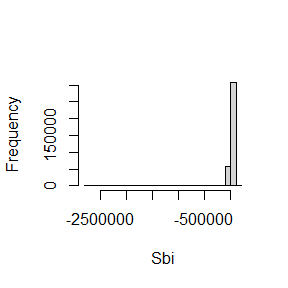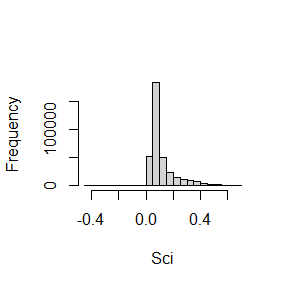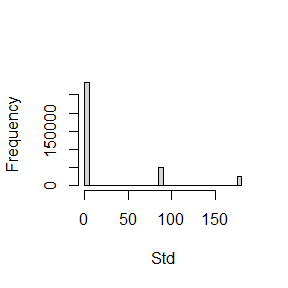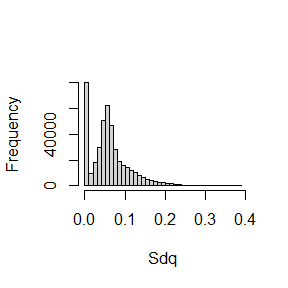The code below visualizes metrics over the entire state in order to capture different aspects of landscape heterogeneity. Individual metrics primarily distinguish managed versus more natural areas; however, some metrics are difficult to interpret, or do not show very much variation over the region. The difficulty of interpreting metrics is a known complicating factor for their use. Others have addressed this issue and linked metrics with known ecosystem features or patch metrics (McGarigal et al., 2009; Kedron et al., 2018).

# New names for plots
plt_names <- data.frame(old = names(data_evi)[3:ncol(data_evi)],
new = c('Sa', 'Sq', 'S10z', 'Sdq', 'Sdq6', 'Sdr',
'Sbi', 'Sci', 'Ssk', 'Sku', 'Sds',
'Sfd', 'Srw', 'Srwi', 'Shw', 'Std', 'Stdi',
'Svi', 'Str (0.2)', 'Str (0.3)', 'Ssc', 'Sv',
'Sp', 'Sk', 'Smean', 'Spk', 'Svk',
'Scl - min (0.2)', 'Scl - max (0.2)',
'Scl - max (0.3)', 'Scl - max (0.3)',
'Sdc 0-5%', 'Sdc 50-55%', 'Sdc 80-85%'))

create_maps <- function(df, r, theme) {
maps_list <- list()
for (i in seq(3, ncol(df))) {
temp <- setValues(r, df[, i])
temp[is.na(r)] <- NA
goodname <- as.character(plt_names$new[plt_names$old == names(df)[i]])
maps_list[[i - 2]] <- rasterVis::levelplot(temp, margin = F,
par.settings = theme,
ylab = NULL, xlab = NULL,
main = goodname)
maps_list[[i - 2]]$par.settings$layout.heights[
maps_list[[i - 2]]$aspect.fill <- TRUE names(maps_list)[i - 2] <- goodname } return(maps_list) } # Create plots of all possible surface gradient metrics that geodiv calculates # for EVI. evi_maps <- create_maps(data_evi, evi_masked, eviTheme) # Create map panels. grid.arrange(grobs = evi_maps[1:12], nrow = 4, ncol = 3) # 850x800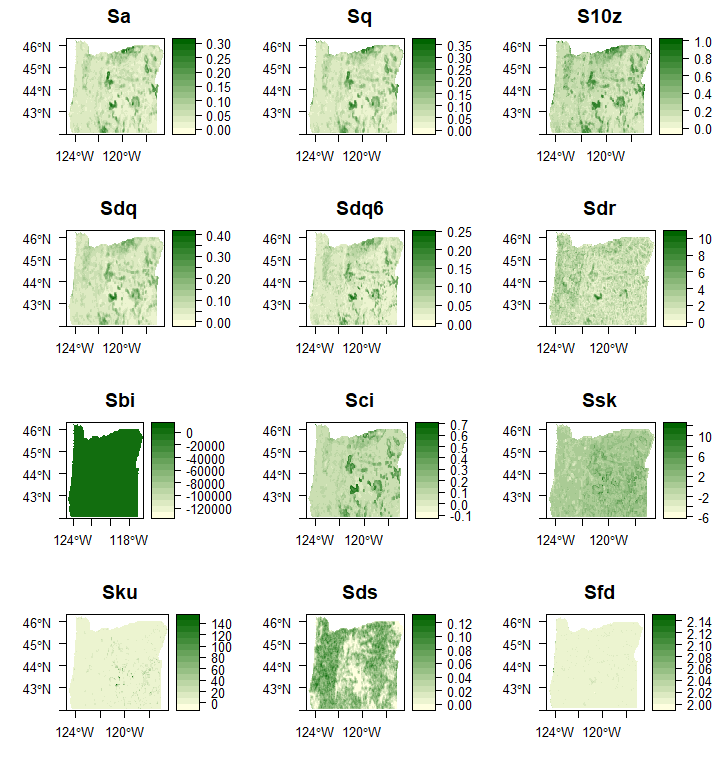grid.arrange(grobs = evi_maps[13:24], nrow = 4, ncol = 3) # 850x800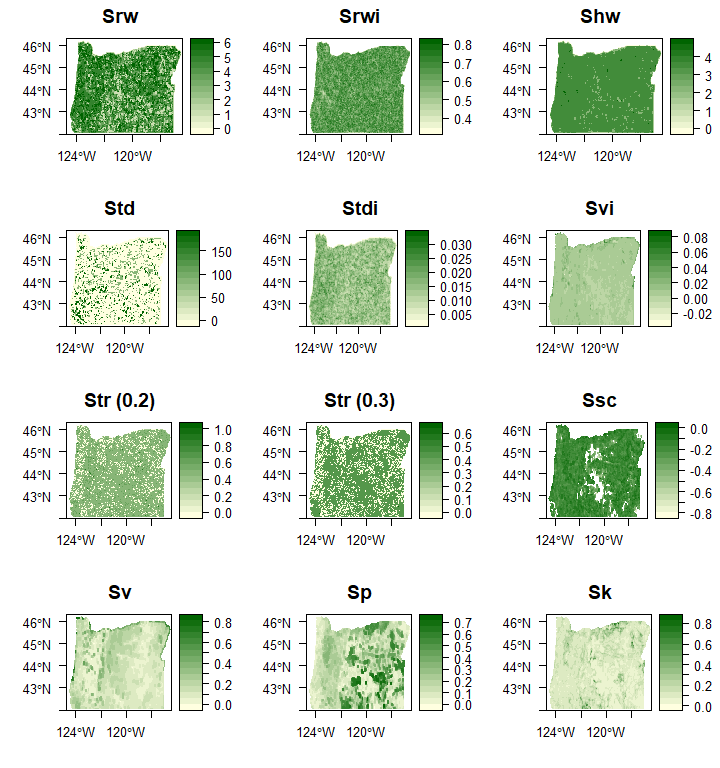grid.arrange(grobs = evi_maps[25:34], nrow = 4, ncol = 3) # 850x800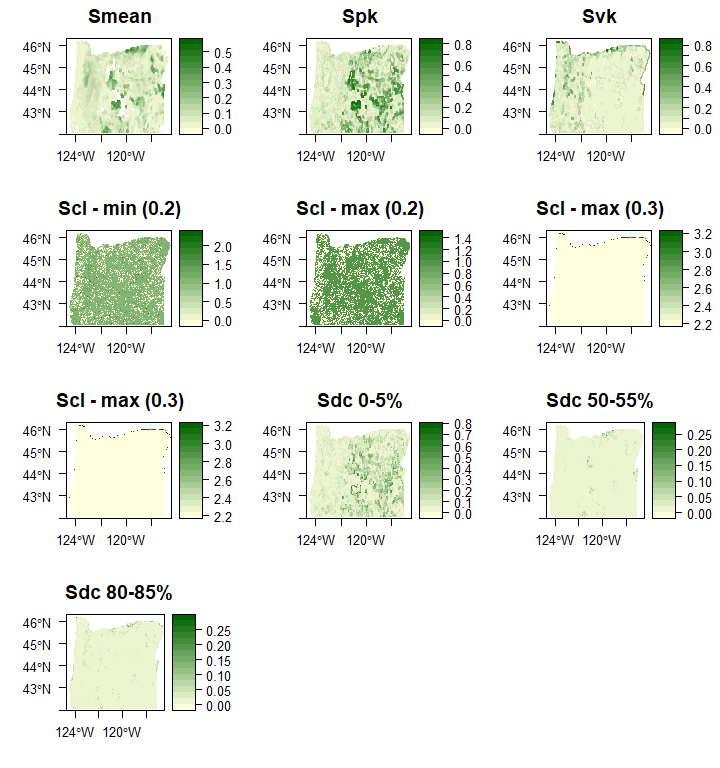Transect analysis In the code below, we examine an example of local correlation and clustering among the surface gradient metrics by extracting values over a horizontal transect across central Oregon. This analysis is meant to demonstrate which metrics tend to represent similar aspects of the landscape, how metrics might be combined to get a more complete representation of the landscape, and ways in which metrics might be further examined prior to an analysis. First, we convert the raw EVI data from the NASA’s MODIS mission to a dataframe and add those raw values to the dataframe containing the gradient surface metrics we’ve calculated across Oregon. # Convert the rasters to dataframe format and add value to dataframe with # metric values. sp_df <- function(r, df) { pixdf <- as.data.frame(as(r, "SpatialPixelsDataFrame")) df$value <- pixdf[, 1]
return(df)
}

data_evi <- sp_df(evi, data_evi)

Now we extract the data along a latitudinal transect going across Oregon.

# Create new dataframe of values along a latitudinal transect.
get_transect <- function(r, df) {
# Crop raster to center transect (+/- 7 pixels North or South).
center_row <- round(nrow(r) / 2)
r_crop <- crop(r, extent(r, center_row - 7, center_row + 7, 1, ncol(r)))

# Get 8th latitudinal coordinate (center latitude) from the cropped raster.
central_y <- unique(coordinates(r_crop)[, 2])

# Get the closest latitude in the dataframe to the central raster coordinate.
central_y <- unique(df$y[near(df$y, central_y, 0.01)])

# Extract mean EVI and elevation values by raster column.
r_means <- colMeans(as.matrix(r_crop), na.rm = TRUE)

# Now limit the dataframe to the central row across the transect.
transect_vals <- df[df$y == central_y,] # Add column means to dataframe. transect_vals$value <- r_means

return(transect_vals)
}

transect_evi <- get_transect(evi, data_evi)

The code below places standardizes all metrics by placing them on the same scale from 0 - 1.

# Get all metrics on same scale (0-1).
scale_mets <- function(df) {
for (i in 3:ncol(df)) {
df[,i] <- (df[, i] - min(df[, i], na.rm = TRUE)) /
(max(df[, i], na.rm = TRUE) - min(df[, i], na.rm = TRUE))
}
return(df)
}

transect_evi <- scale_mets(transect_evi)

Clustering analysis over transect

For the transect analysis, we will perform hierarchical clustering on metric values using the function ‘eclust’ in the package factoextra (Kassambara & Mundt, 2020). First, some additional data wrangling is required to prepare the data for the clustering analysis below.

# Remove NA values from the metric columns.
rm_nas <- function(df) {
for (i in 3:ncol(df)) {
df <- df[!is.na(df[, i]),]
}
return(df)
}

transect_evi <- rm_nas(transect_evi)

The code below performs the clustering analysis on the surface gradient metrics. We first determine the optimal number of clusters by examining the gap statistic, and then plot the clustered variables to see the relationships among them.

### Plot optimal number of clusters
plot_gap <- function(df) {
# enhanced k-means clustering
res.km <- clusGap(t(df)[3:(ncol(df) - 1), ], stats::kmeans, K.max = 10,
B = 100, nstart = 25)
# gap statistic plot
fviz_gap_stat(res.km)
}

plot_gap(transect_evi)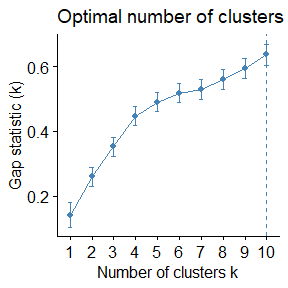### Dendrogram and scatterplot of clusters
get_clusters <- function(df, nclust) {
# Enhanced hierarchical clustering using optimal # of clusters.
res.hc <- eclust(t(df)[3:(ncol(df) - 1),],
"hclust", k = nclust)

return(res.hc)
}

plot_dendrogram <- function(res.hc, nclust){
# Plot colors
plt_cols <- c('lightgoldenrod1', 'lightblue', 'grey', 'lightsteelblue4')

# Dendrogram plot
fviz_dend(res.hc, rect = FALSE, k_colors = plt_cols[1:nclust],
lwd = 1, label_cols = 'black', cex = 0.8, main = "", ylab = "",
type = 'rectangle', horiz = TRUE, labels_track_height = 14) +
theme(axis.text.y = element_blank(), axis.ticks = element_blank())
}

res.hc_evi <- get_clusters(transect_evi, nclust = 3)

plot_dendrogram(res.hc_evi, nclust = 3)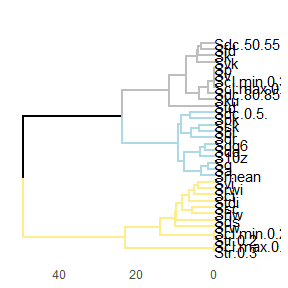Now we generate plots that show the EVI surface gradient metrics along the Oregon state transect.

First, the data have to be gathered into a longer format for this visualization.

# Create gathered (long) version of dataframe for the clustering analysis.
gather_data <- function(df) {
df <- df %>% gather(key = 'var', value = 'value',
names(df[, seq(3, ncol(df))]))

# Order variables.
df <- df[order(df$var),] return(df) } gathered_evi <- gather_data(transect_evi) Now we can plot the metrics along the transect, labeling the cluster. # Plot metrics along transect, with cluster labeled. plot_transect_mets <- function(df, res.hc, varname) { # Map colors to cluster or variable names. col_map <- c("1" = "lightgoldenrod1", "2" = "lightblue", "3" = "grey", "4" = "lightsteelblue4", "EVI" = "white", "Elev" = "white") # Create a dataframe to match variable names with cluster number. clust_df <- data.frame(var = res.hc$labels, clust = res.hc$cluster) clust_df <- clust_df[order(clust_df$clust),]

# Convert var to character.
clust_df$var <- as.character(clust_df$var)

# Join cluster number with main dataframe to get cluster labels for plotting.
df <- left_join(df, clust_df, by = 'var')

# Anything not labeled with a cluster (i.e., the actual value) gets labeled.
df$clust[is.na(df$clust)] <- varname

# Change 'value' label to actual variable name.
df$var[df$var == 'value'] <- varname

# Convert cluster names to factors and match with colors.
df$clust <- as.factor(df$clust)
df$var <- factor(df$var, levels = c(clust_df$var, varname)) cols_to_use <- col_map[names(col_map) %in% df$clust]

ggplot(df, aes(x = x, y = value)) +
geom_rect(aes(xmin = -Inf, xmax = Inf, ymin = -Inf, ymax = Inf,
fill = clust)) +
geom_line(lwd = 0.7) +
xlab('Longitude') +
facet_grid(var~., switch = 'y') +
scale_fill_manual(values = cols_to_use, name = 'Cluster') +
theme_bw() +
theme(axis.title.y = element_blank(),
axis.text.y = element_blank(),
strip.text.y.left = element_text(face = 'bold', size = 11, angle = 0),
legend.position = 'none',
axis.title.x = element_text(face = 'bold', size = 11))
}

plot_transect_mets(gathered_evi, res.hc_evi, "EVI")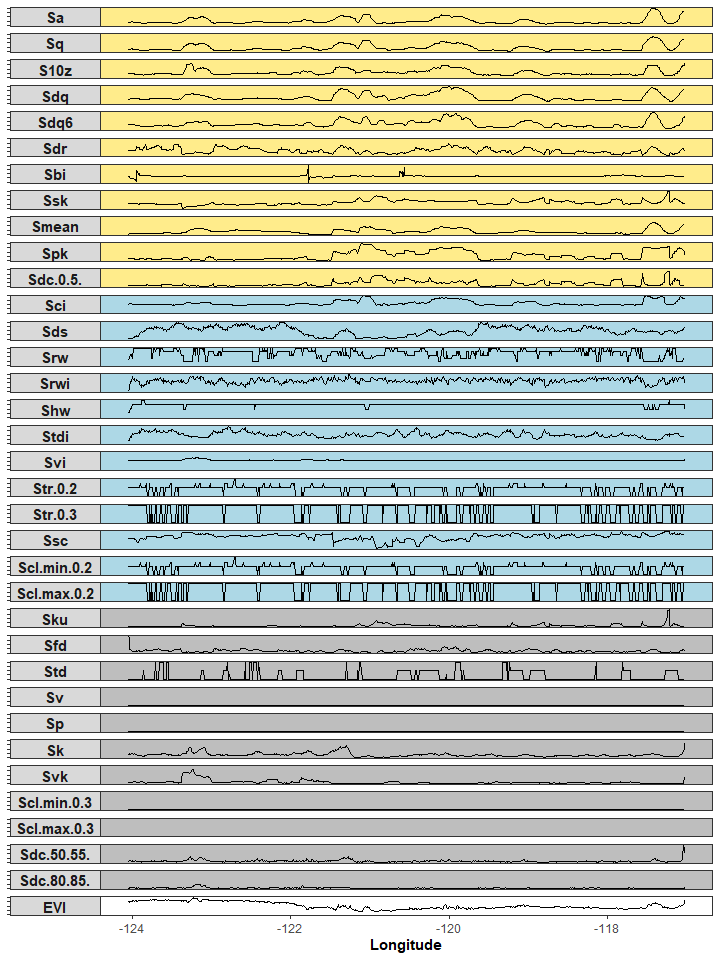Summary of transect cluster analysis

Overall trends along the transect were similar among metrics, with more variation at smaller intervals. Using hierarchical clustering, we found four clusters of metrics for elevation, and three for EVI. The metrics fell into different combinations based on the variable considered (elevation or EVI). For example, Sdq6 and S10z grouped together for both variables, but Std and Srw were in the same group for elevation, and different groups for EVI.

Principal Components Analysis (PCA)

Next, we will determine the statewide elevation and EVI variance explained by metrics using PCA. First, we need to get the data ready for the PCA. In the code below, we remove several variables due to their large number of NA values, caused either by windows containing too few values, or windows lacking ‘peaks’ or ‘valleys’ (pixels surrounded by lower or higher values, respectively). After cleaning the data, 21 metrics remain in the analysis.

# Get data ready for PCA by removing NA values.
clean_data <- function(df) {
# Remove columns with very large numbers of NAs.
NAs <- sapply(df, function(x) sum(is.na(x)))
rm_cols <- which(NAs >= 90000)
df <- df[, -rm_cols]
# Remove NAs from remaining columns.
df <- na.omit(df)
return(df)
}

data_evi_noNA <- clean_data(data_evi)

In the code below, the PCA is performed with the remaining metrics using the ‘prcomp’ function in the stats package.

# Calculate the principal components.
evi_prc <- prcomp(data_evi_noNA[,3:23], center = TRUE, scale = TRUE)
summary(evi_prc)
#> Importance of components:
#>                           PC1    PC2     PC3     PC4     PC5     PC6     PC7
#> Standard deviation     2.7279 2.0779 1.44798 1.16300 1.00042 0.99381 0.92085
#> Proportion of Variance 0.3543 0.2056 0.09984 0.06441 0.04766 0.04703 0.04038
#> Cumulative Proportion  0.3543 0.5599 0.65979 0.72420 0.77186 0.81889 0.85927
#>                            PC8     PC9    PC10    PC11    PC12    PC13   PC14
#> Standard deviation     0.86682 0.77264 0.62066 0.59155 0.47773 0.45224 0.3460
#> Proportion of Variance 0.03578 0.02843 0.01834 0.01666 0.01087 0.00974 0.0057
#> Cumulative Proportion  0.89505 0.92347 0.94182 0.95848 0.96935 0.97909 0.9848
#>                           PC15    PC16    PC17    PC18    PC19   PC20    PC21
#> Standard deviation     0.31487 0.27919 0.25551 0.20093 0.13248 0.1214 0.06617
#> Proportion of Variance 0.00472 0.00371 0.00311 0.00192 0.00084 0.0007 0.00021
#> Cumulative Proportion  0.98951 0.99322 0.99633 0.99825 0.99909 0.9998 1.00000

Now let’s look at some diagnostic plots for the principal components. Scree plots indicate the importance of the principal components with a broken stick criterion. The point at which the scree plot curve crosses the broken stick model distribution, which we will plot in red, is considered to indicate the maximum number of components to retain.

# Take a look at the components using a screeplot.
plot_scree <- function(pc_dat) {
screeplot(pc_dat, type = "l", npcs = 15,
main = "Screeplot of the first 10 PCs")
abline(h = 1, col = "red", lty = 5)
legend("topright", legend = c("Eigenvalue = 1"),
col = c("red"), lty = 5, cex = 0.6)
}

plot_scree(evi_prc)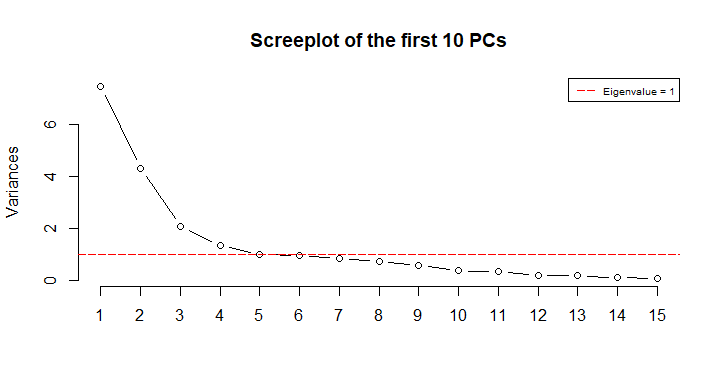We can also take a look at the components for elevation and EVI to see how much variance the surface metrics explain with cumulative variance plots.

# Look at how much variance is explained using a cumulative variance plot.
plot_cvar <- function(pc_dat) {
# Get cumulative variance explained.
cumpro <- summary(pc_dat)$importance[3, ][1:16] # Create plot of cumulative variance, marking the 5th component as the cutoff. plot(cumpro, xlab = "PC #", ylab = "Amount of explained variance", main = "Cumulative variance plot") abline(v = 5, col = "blue", lty = 5) abline(h = cumpro, col = "blue", lty = 5) legend("topleft", legend = c("Cut-off @ PC5"), col = c("blue"), lty = 5, cex = 0.6) } plot_cvar(evi_prc)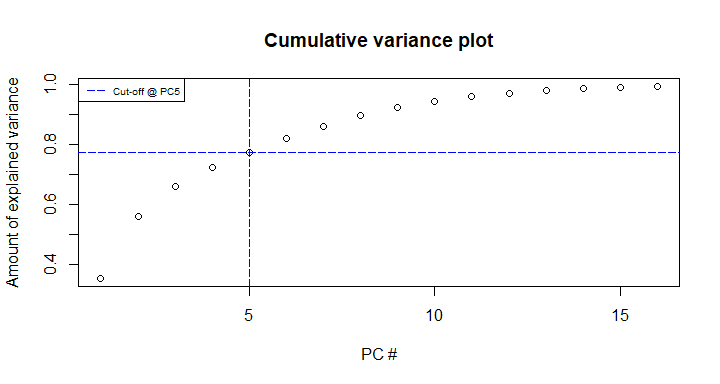For EVI, the first 5 principal components explain >70% of the variation. In the code below, we map the components to see if there are any spatial patterns readily identifiable. # Map components across state. map_comps <- function(pc_dat, noNA_df, full_df, r, theme) { # Add pc values to no-NA dataframe. for (i in 1:5) { colname <- paste0('prc', i) noNA_df[, colname] <- pc_dat$x[, i]
}

# Add PCA results back to full raster dataframe.
full_dat <- full_df %>% left_join(noNA_df)
# Cut to only the prc columns.
full_dat <- full_dat[, grep('prc', names(full_dat))]

# Create rasters and maps with principle component values.
out_maps <- list()
for (i in 1:5) {
new_rast <- setValues(r, full_dat[, i])
pc_map <- rasterVis::levelplot(new_rast, margin = F,
par.settings = theme,
ylab = NULL, xlab = NULL,
main = paste0('PC', i))
pc_map$par.settings$layout.heights[c( 'bottom.padding',
pc_map$aspect.fill <- TRUE out_maps[[i]] <- pc_map } # Plot in a grid. grid.arrange(grobs = out_maps, nrow = 2, ncol = 3) } map_comps(evi_prc, data_evi_noNA, data_evi, evi, eviTheme) #> Joining, by = c("x", "y", "Sa", "Sq", "S10z", "Sdq", "Sdq6", "Sdr", "Sbi", "Sci", "Ssk", "Sku", "Sds", "Std", "Svi", "Sv", "Sp", "Sk", "Spk", "Svk", "Sdc.0.5.", "Sdc.50.55.", "Sdc.80.85.", "value")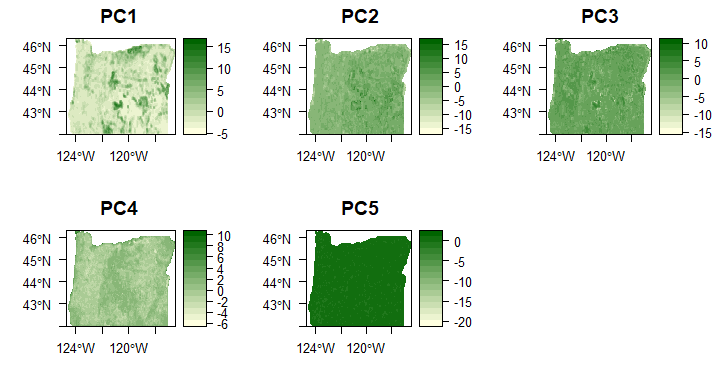What are the principal component loadings? # Plot principal component loadings. plot_loadings <- function(pc_dat) { # Get rotation for top 5 components. loadings <- pc_dat$rotation[, 1:5]

rel <- as.data.frame(rel)
# Get good variable names (from dataframe created earlier).
rel$var <- plt_names$new[match(rownames(rel), plt_names$old)] # Create importance plots. imp_plts <- list() for (i in 1:5) { temp <- rel # Determine whether component loading is postive or negative. temp$sign <- factor(sapply(loadings[, i], FUN = function(x) x / abs(x)),
levels = c(-1, 1))

temp <- temp[order(temp[, i]),]

temp$var <- factor(temp$var, levels = temp\$var)

temp_plt <- ggplot(temp, aes(x = temp[, i], y = var)) +
geom_point(size = 3, aes(pch = sign)) +
scale_shape_manual(name = element_blank(),
breaks = c(1, -1),
values = c(19, 8),
labels = c("Positive", "Negative")) +
xlab(paste0('PC', i)) +
ylab('Metric') +
theme_bw() +
theme(panel.grid.minor = element_blank(),
legend.justification = c(1, 0),
legend.position = c(1, 0),
legend.background = element_blank(),
legend.text = element_text(size = 12),
axis.title = element_text(size = 12))

imp_plts[[i]] <- temp_plt
}

# Return grid of first three components.
grid.arrange(grobs = imp_plts[1:3], ncol = 3)
}